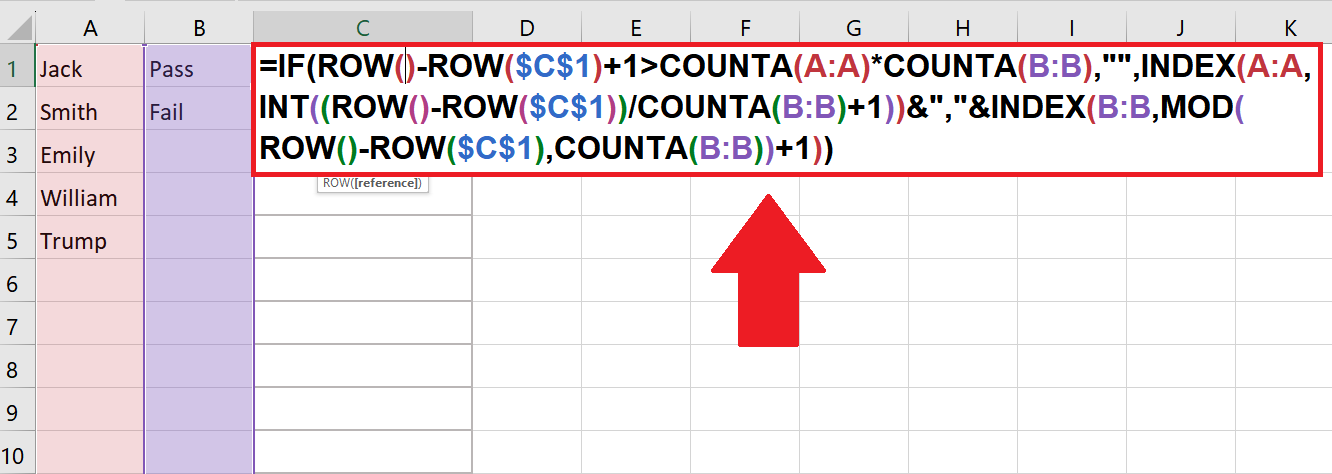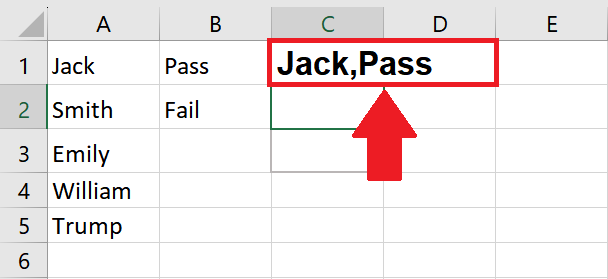# How to calculate permutations in Excel

In this tutorial, the dataset is of two columns and we have to show its permutation. The first column contains the names of students, while the second column indicates their status as “Fail” or “Pass” based on certain conditions. By using permutations in this context, we get all possible arrangements of students’ names while considering their pass or fail status.Permutation refers to a mathematical concept related to arranging a set of items in a particular order. In Excel, permutations are often used in various scenarios, such as data analysis, probability calculations, and combinatorial problems. They can be utilized to generate unique data combinations or to calculate the number of possible arrangements for a given set of items.

### Step 1 – Select the Column– First, select the cell where you want to get permutation

### Step 2 – Type Formula– Now type the formula in the first cell of the selected column as follows:

=IF(ROW()-ROW(\$C\$1)+1>COUNTA(A:A)*COUNTA(B:B),””,INDEX(A:A,INT((ROW()-ROW(\$C\$1))/COUNTA(B:B)+1))&”,”&INDEX(B:B,MOD(ROW()-ROW(\$C\$1),COUNTA(B:B)+1))

– The ROW() function retrieves the current row number.
– ROW(\$C\$1) is subtracted to adjust the calculation based on the reference point.
– COUNTA(A: A) counts the number of non-empty cells in column A (names).
– COUNTA(B: B) counts the number of non-empty cells in column B (conditions).
– The formula checks if the current row number exceeds the total number of permutations.
– If the condition is true, an empty string is returned, indicating the end of the permutations.
– The INT() function calculates the row number for the names by dividing the adjusted row number by the number of conditions.
– The INDEX(A: A, …) retrieves the name from column A based on the calculated row number.
– The MOD() function calculates the row number for the conditions by dividing the remainder of the adjusted row number by the number of situations.
– The INDEX(B:B, …) retrieves the condition from column B based on the calculated row number.
– The name and condition are concatenated using the & operator and separated by a comma.
– The resulting permutation is displayed in the corresponding cell.

### Step 3 – Press the Enter Key– Now press the enter key and you will get the result

### Step 4 – Drag the Cell– After getting the result in the first cell, drag the cell to the required location, to get the complete permutations